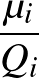Next: Point Group Determination Up: OPTIM User Guide Previous: Running OPTIM   Contents

# Step Scaling Criteria

Once an eigenvector-following step of whatever type has been calculated it will generally need to be scaled down. Five methods are available for doing this, as set by the keyword SCALE.

For SCALE 0 the `step length' is taken to be the total step length in the multidimensional space of Cartesian coordinates. For SCALE 1 we scale according to the largest displacement in Cartesian coordinates. For SCALE 2 we scale according to the largest displacement in the Hessian eigenvector basis. For SCALE 3 we scale according to the total step size called for by the steepest-descent routine. The default for all other SEARCH types is now SCALE 10 for which a different trust ratio is calculated for each eigendirection and used to adjust the maximum allowed step for each eigenvector. This is described in more detail below.

SCALE 10 employs a trust radius whose default value is 4.0. This value can be changed using the keyword TRAD. Appropriate values for the maximum initial step size and the trust radius for different systems may need to be found by experimentation. After the first step the program compares the predicted value of the Hessian eigenvalue for a given eigendirection, as calculated by finite difference of the present and previous component of the gradient in this direction, with the actual eigenvalue. The trust ratio for a particular eigendirection is the modulus of this predicted value minus the actual value, divided by the actual value. If the trust ratio is less than the trust radius then the maximum step allowed in this direction is increased by a factor of 1.1 (up to a maximum of 0.5); if it is more than the trust radius the maximum allowed step in this direction is divided by 1.1 (down to a minimum allowed value of 0.005). The maximum allowed step for each direction is initialised at the value read from the odata file.

The trust ratio is not used in a transition state search for the uphill direction if the corresponding eigenvalue is positive; the step size is instead set to the value of the MAXMAX parameter.

This trust ratio approach assumes that all the eigenvectors are in correspondence for the present and previous geometries when they are arranged according to the eigenvalues in ascending or descending order. When large steps are being taken some eigendirections are likely to cross over, and it might be better to use an overlap criterion for all the present and previous eigenvectors. However, this has not proved to be necessary to date.

Scaling of the above sort does not apply in :BFGS minimisations, although the trust radius approach described above for SCALE 10 is used for the eigenvector-following step in hybrid transition state searches.

For SEARCH 0, 2, 3 and 4 OPTIM detects convergence to stationary points with the wrong Hessian index and steps away from them. The test is triggered when the RMS force falls below the value specified by PUSHCUT (default 10-5) in the prevailing units. The test is only applied at the first step and every fourth step. If a transition state search converges to (or starts from) a minimum a step is taken in the direction specified by MODE of magnitude PUSHOFF. If PUSHOFF has not been set then the displacement is one tenth of the current maximum step size allowed for the eigenmode in question. If a transition state search converges to (or starts from) a higher index saddle then the action depends upon the value of MODE. For MODE 0 displacements are taken for all the eigenmodes with negative eigenvalues except for the one with the most negative eigenvalue. The magnitude of the displacement is determined in the same way as above. For MODE n0 a displacement is applied only along the specified value of MODE. For search types 0 and 3 similar action is taken. If the search converges to (or starts from) a stationary point that is not a minimum then if MODE 0 displacements are applied for all the eigenmodes with negative eigenvalues. If MODE n0 then a displacement is only applied in the direction specified by MODE.Next: Point Group Determination Up: OPTIM User Guide Previous: Running OPTIM   Contents